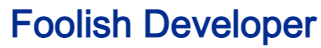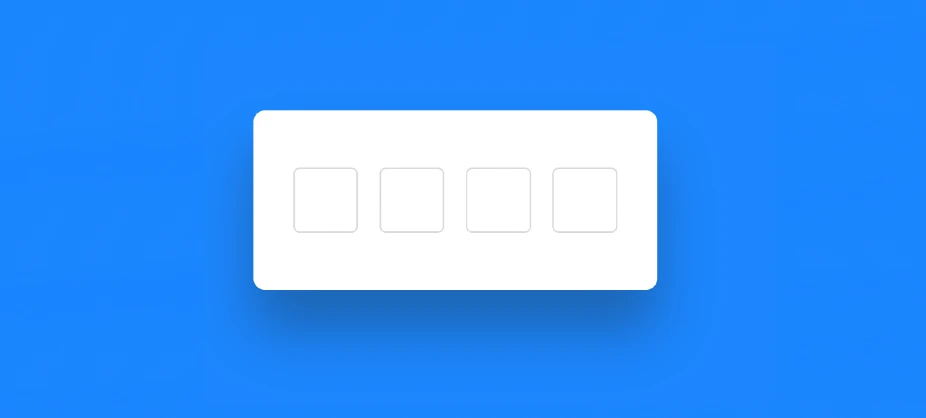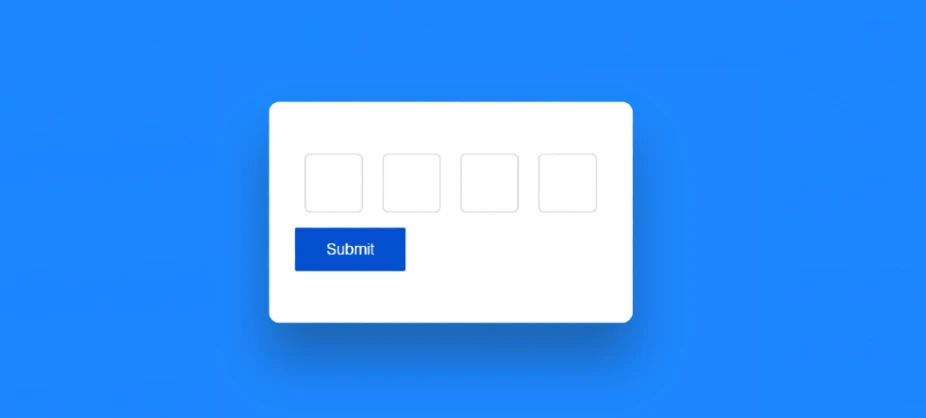Foolish Developer# Complete Guide about web designing - Foolish DeveloperDo you want to create OTP Input Field using HTML css and javascript? In this article, you will know how to make OTP Page With Autofocus Input Fields using JavaScript.This is a simple UI project for beginners. OTP/PIN Input Field is used in many places. Although it is not connected to the server. This is just a design. But I have made this OTP PIN Input Field more advanced by JavaScript. Various disclaimers and conditions are added here.

## OTP input field html css javascript

We see OTP Screen UI in various places. In this tutorial I will create such an OTP HTML Input. Next time I’ll show how to connect it to the server via PHP.

Here I have created the basic structure of this Simple OTP Input Field with html. Designed it with css and activated it with javascript.

See the Pen OTP Input Field using JavaScript by Ground Tutorial (@groundtutorial) on CodePen.

As you can see above I have created a box on a web page which will serve as the basic structure of this project (creating otp pin input field using html css and javascript). The box has four small input boxes where you can input numbers.

Once the number is entered in the input box, it cannot be edited and after entering the number in each input box, the submit button can be seen here.

## how to create otp input field using html css and javascript

Now if you want to build(How To Create OTP Input Field Using HTML , CSS & Javascript) this project then follow the tutorial below. Here you will get complete information step by step tutorial and complete source code.

If you only want to download this Otp Input Field Html Css Javascript source code then you can use the download button below the article.

But if you are a beginner then I request you to follow the complete tutorial and create this OTP Page With Autofocus Input Fields.

### Step 1: Basic Structure of OTP Input Field

First I created a box on the web page using the following html. Here I have used blue color in the background of the web page. The basic structure of OTP Input Field is width: 28em and background-color: #ffffff is used.

```				```
<div class="container">

</div>
```
```
```				```
* {
margin: 0;
box-sizing: border-box;
}
body {
height: 100vh;
background: rgb(28, 134, 255)
}
```
```
```				```
.container {
width: 28em;
background-color: #ffffff;
position: absolute;
transform: translate(-50%, -50%);
top: 50%;
left: 50%;
box-shadow: 0 45px 60px rgba(30, 22, 1, 0.3);
}
```
```### Step 2: Create space to input OTP

The input function of html is used to create the input box within this OTP Screen UI. Here I have used type=”number” so you can input only numbers. Then the input boxes are designed with some CSS.

```				```
<div class="inputfield">
<input type="number" maxlength="1" class="input" disabled />
<input type="number" maxlength="1" class="input" disabled />
<input type="number" maxlength="1" class="input" disabled />
<input type="number" maxlength="1" class="input" disabled />
</div>
```
```
```				```
.inputfield {
width: 100%;
display: flex;
justify-content: space-around;
}
.input {
height: 3em;
width: 3em;
outline: none;
text-align: center;
font-size: 1.5em;
background-color: #ffffff;
outline: none;
/*Hide number field arrows*/
-moz-appearance: textfield;
}
```
```

Now tried to make it more beautiful by using :disabled and :focus. Define what happens when the input box is disabled or :focused.

```				```
input[type="number"]::-webkit-outer-spin-button,
input[type="number"]::-webkit-inner-spin-button {
-webkit-appearance: none;
margin: 0;
}

.input:disabled {
color: #89888b;
}
.input:focus {
border: 3px solid #ffb800;
}
```
```### Step 3: Create submit button in OTP Input Field

Now create a submit button. Clicking on this button will submit the inputted information. The button is created using the button function of html.

```				```
<button class="hide" id="submit" onclick="validateOTP()">Submit</button>
```
```
```				```
#submit {
background-color: #044ecf;
border: none;
outline: none;
font-size: 1.2em;
color: #ffffff;
margin: 1em auto 0 auto;
cursor: pointer;
}
```
``````				```
.show {
display: block;
}
.hide {
display: none;
}
```
```

### Step 4: Activate OTP Verification Form by JavaScript

Now it’s time to implement the html OTP Input Field with JavaScript. Used a lot of javascript here. But if you know basic javascript then you can create this OTP Verification Form in HTML CSS very easily.

```				```
//Initial references
const input = document.querySelectorAll(".input");
const inputField = document.querySelector(".inputfield");
const submitButton = document.getElementById("submit");
let inputCount = 0,
finalInput = "";

//Update input
const updateInputConfig = (element, disabledStatus) => {
element.disabled = disabledStatus;
if (!disabledStatus) {
element.focus();
} else {
element.blur();
}
};

input.forEach((element) => {
e.target.value = e.target.value.replace(/[^0-9]/g, "");
let { value } = e.target;

if (value.length == 1) {
updateInputConfig(e.target, true);
if (inputCount <= 3 && e.key != "Backspace") {
finalInput += value;
if (inputCount < 3) {
updateInputConfig(e.target.nextElementSibling, false);
}
}
inputCount += 1;
} else if (value.length == 0 && e.key == "Backspace") {
finalInput = finalInput.substring(0, finalInput.length - 1);
if (inputCount == 0) {
updateInputConfig(e.target, false);
return false;
}
updateInputConfig(e.target, true);
e.target.previousElementSibling.value = "";
updateInputConfig(e.target.previousElementSibling, false);
inputCount -= 1;
} else if (value.length > 1) {
e.target.value = value.split("");
}
});
});

if (inputCount > 3) {
submitButton.classList.remove("hide");
if (e.key == "Backspace") {
finalInput = finalInput.substring(0, finalInput.length - 1);
updateInputConfig(inputField.lastElementChild, false);
inputField.lastElementChild.value = "";
inputCount -= 1;
}
}
});

const validateOTP = () => {
};

//Start
const startInput = () => {
inputCount = 0;
finalInput = "";
input.forEach((element) => {
element.value = "";
});
updateInputConfig(inputField.firstElementChild, false);
};

```
```I hope you learned from the above tutorial how I created the project(OTP Screen UI using HTML, CSS & JS). If you want to know more about JavaScript tutorials, you can search for my blog.

Please comment on how you like this Javascript OTP Input Field. Use the download button below to get the source code of the OTP pin input field.

From this tutorial you can create OTP Input Field very easily. And it will be best for you because the code used here is very simple so you can understand very easily. Also here you will get the complete source code.